# SMHS SciVisualization SOCR Excel R Charts

## SOCR Charts

• URL: http://socr.umich.edu/html/cha/ (Java applet)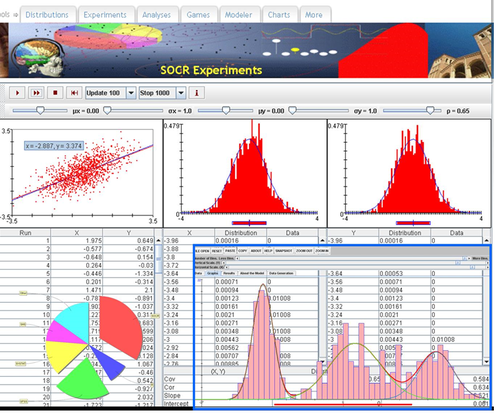## Excel Charts

MS Excel provides a large number of charts that can be used to quickly and effectively render complex multivariate data. For instance, the table below contains the principal component analysis (PCA) of 50 derived neuroimaging biomarkers (region of interest (ROI) by shape morphometry metric). The loadings of these 50 variables on the top 5 (most significant) principal component directions are shown in the table. Experiment with effective visualizations of these data.

Hemi ROI measure ROI_Measure Dim.1 Dim.2 Dim.3 Dim.4 Dim.5
L insular AvgMeanCurvature L_insular_cortex_AvgMeanCurvature 0.72 0 0.06 0.06 0
L insular ComputeArea L_insular_cortex_ComputeArea 0.77 0.06 0.04 0.01 0
L insular Volume L_insular_cortex_Volume 0.72 0.09 0.04 0.03 0.01
L insular ShapeIndex L_insular_cortex_ShapeIndex 0.46 0.06 0.01 0.02 0.01
L insular Curvedness L_insular_cortex_Curvedness 0.78 0 0.05 0.03 0.01
R insular AvgMeanCurvature R_insular_cortex_AvgMeanCurvature 0.79 0 0.03 0.08 0
R insular ComputeArea R_insular_cortex_ComputeArea 0.79 0.09 0.03 0.01 0
R insular Volume R_insular_cortex_Volume 0.73 0.11 0.03 0.03 0
R insular ShapeIndex R_insular_cortex_ShapeIndex 0.27 0.17 0 0.07 0
R insular Curvedness R_insular_cortex_Curvedness 0.84 0.02 0.03 0.01 0
L cingulate AvgMeanCurvature L_cingulate_gyrus_AvgMeanCurvature 0.72 0 0.05 0.02 0.02
L cingulate ComputeArea L_cingulate_gyrus_ComputeArea 0.74 0.05 0.06 0.04 0.01
L cingulate Volume L_cingulate_gyrus_Volume 0.69 0.08 0.05 0.05 0.01
L cingulate ShapeIndex L_cingulate_gyrus_ShapeIndex 0.53 0 0.05 0 0.03
L cingulate Curvedness L_cingulate_gyrus_Curvedness 0.7 0.01 0.05 0.04 0.03
R cingulate AvgMeanCurvature R_cingulate_gyrus_AvgMeanCurvature 0.6 0 0.02 0.03 0.01
R cingulate ComputeArea R_cingulate_gyrus_ComputeArea 0.73 0.06 0.04 0.03 0.01
R cingulate Volume R_cingulate_gyrus_Volume 0.68 0.09 0.04 0.04 0.01
R cingulate ShapeIndex R_cingulate_gyrus_ShapeIndex 0.56 0.01 0.05 0 0.01
R cingulate Curvedness R_cingulate_gyrus_Curvedness 0.25 0 0.01 0.04 0
L caudate AvgMeanCurvature L_caudate_AvgMeanCurvature 0.52 0 0.05 0 0.01
L caudate ComputeArea L_caudate_ComputeArea 0.51 0.09 0.03 0.04 0.02
L caudate Volume L_caudate_Volume 0.44 0.09 0.03 0.06 0.03
L caudate ShapeIndex L_caudate_ShapeIndex 0.2 0.03 0.04 0.04 0
L caudate Curvedness L_caudate_Curvedness 0.51 0.12 0.02 0.01 0.01
R caudate AvgMeanCurvature R_caudate_AvgMeanCurvature 0.68 0.04 0.04 0.02 0
R caudate ComputeArea R_caudate_ComputeArea 0.67 0.17 0.03 0.02 0.01
R caudate Volume R_caudate_Volume 0.61 0.16 0.02 0.03 0.01
R caudate ShapeIndex R_caudate_ShapeIndex 0.18 0.02 0.03 0.11 0
R caudate Curvedness R_caudate_Curvedness 0.65 0.19 0.01 0 0
L putamen AvgMeanCurvature L_putamen_AvgMeanCurvature 0.62 0 0.04 0.03 0.02
L putamen ComputeArea L_putamen_ComputeArea 0.56 0.05 0.04 0.03 0.05
L putamen Volume L_putamen_Volume 0.52 0.07 0.04 0.05 0.05
L putamen ShapeIndex L_putamen_ShapeIndex 0.06 0.13 0 0.15 0
L putamen Curvedness L_putamen_Curvedness 0.64 0.11 0.03 0.01 0.03
R putamen AvgMeanCurvature R_putamen_AvgMeanCurvature 0.62 0 0.07 0.04 0.01
R putamen ComputeArea R_putamen_ComputeArea 0.66 0.08 0.03 0.01 0.03
R putamen Volume R_putamen_Volume 0.64 0.12 0.03 0.02 0.03
R putamen ShapeIndex R_putamen_ShapeIndex 0.15 0.24 0 0.08 0.03
R putamen Curvedness R_putamen_Curvedness 0.65 0.05 0.05 0 0.02
L hippocampus AvgMeanCurvature L_hippocampus_AvgMeanCurvature 0.78 0 0.01 0.04 0
L hippocampus ComputeArea L_hippocampus_ComputeArea 0.75 0.07 0.01 0 0.02
L hippocampus Volume L_hippocampus_Volume 0.72 0.09 0.01 0.01 0.01
L hippocampus ShapeIndex L_hippocampus_ShapeIndex 0.45 0.17 0 0.04 0.02
L hippocampus Curvedness L_hippocampus_Curvedness 0.79 0.03 0.01 0 0.02
R hippocampus AvgMeanCurvature R_hippocampus_AvgMeanCurvature 0.72 0 0 0.1 0.01
R hippocampus ComputeArea R_hippocampus_ComputeArea 0.71 0.09 0 0 0.05
R hippocampus Volume R_hippocampus_Volume 0.68 0.1 0 0 0.04
R hippocampus ShapeIndex R_hippocampus_ShapeIndex 0.37 0.18 0 0.02 0.03
R hippocampus Curvedness R_hippocampus_Curvedness 0.77 0.03 0 0.02 0.04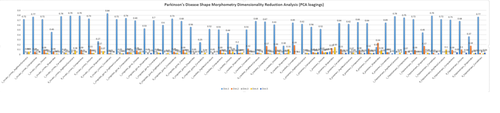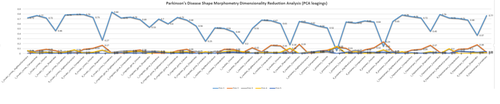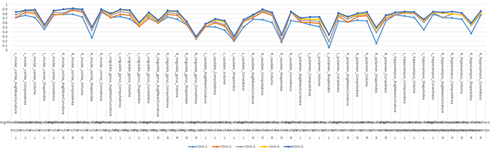## R-Charts

There are 100’s of packages and 1,000 of different charts, plots and graphs that can be generated using R. Such interactive visualizations enable deeper exploration of data, models and results. JavaScript libraries, e.g., D3, provide advantages for data visualization as these involve HTML5 and are easily shareable online. The R community is developing R interfaces to some popular JavaScript libraries to allow users to create interactive visualizations without detailed knowledge of JavaScript.

### Examples of powerful R interactive visualization packages

ggplot2http://ggplot2.org

ggvis – interactive plots extending the static ggplot2 charts, http://ggvis.rstudio.com

rCharts – R interface to multiple JavaScript charting libraries, http://rcharts.io

plotly – transforming ggplot2 charts into interactive plots, https://plot.ly/r

HTMLWidgets

o leaflet – library for creating dynamic maps, supports panning and zooming, annotations, markers, polygons, etc. http://www.htmlwidgets.org/showcase_leaflet.html

o dygraphs – provides mechanism for charting time-series data, supports interactive navigation features including series/point highlighting, zooming, and panning, http://www.htmlwidgets.org/showcase_dygraphs.html

o networkD3 – library for creating D3 network graphs including force directed networks, Sankey diagrams, and Reingold-Tilford tree networks, http://www.htmlwidgets.org/showcase_networkD3.html

o DataTables – displays R matrices or data frames as interactive HTML tables that support filtering, pagination, and sorting, http://www.htmlwidgets.org/showcase_datatables.html

o Rthreejs – features 3D scatterplots and globes based on WebGL, http://www.htmlwidgets.org/showcase_threejs.html

• Other R graphic examples

o To write out plots out to file use:

```# pdf() command all graphs are redirected to test.pdf.  Also works with other common formats:  jpeg, png, ps, tiff.
pdf("C:\\Users\\Dinov\\Desktop\\test.pdf"); plot(1:100, 1:100); dev.off()
# Generates Scalable Vector Graphics (SVG) that can be edited by vector graphics software
svg("test.svg"); plot(1:100, 1:100); dev.off()
```

### Paired ScatterPlots

```set.seed(100)
x <- matrix(runif(50), ncol=5, dimnames=list(letters[1:10], LETTERS[1:5]))
describe(x)    # library("Hmisc")
plot(x[,1], x[,2], pch=20, col="red", main="Symbols and Labels")
text(x[,1]+0.03, x[,2], rownames(x))
```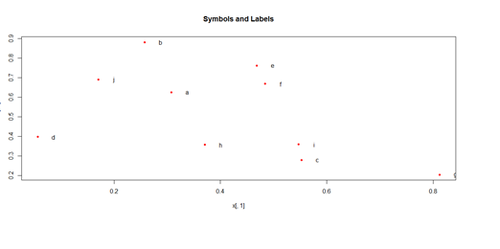```pairs(x)
```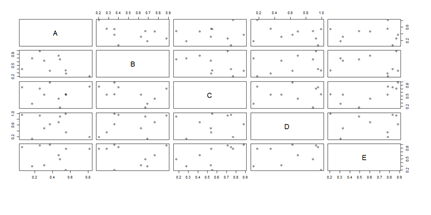Another way to generate scatterplots is by using ggplot:

```# library(ggplot2)
x <- sample(1:20, 20); y <- sample(1:20, 20); cat <- rep(c("A", "B", "C", "D"), 5)
#vs. cat <- rep(c("A", "B", "C", "D"), each=5)
plot.1 <- qplot(x, y, geom="point", size=5*x, color=cat, main="GGplot with Relative Dot Size and Color") + theme(legend.position = "topleft")
print(plot.1)
```
```# Use Case-Studies: https://umich.instructure.com/courses/38100/files/folder/Case_Studies
#  Case_03_MentalHealthServicesSurvey
attach(data1)
# library("Hmisc")
describe(data1)
```
```plot(data1[,3], data1[,4], pch=20, col="red", main="Symbols and Labels")
# text(data1 [,3]+0.03, data1 [,4], rownames(data1))
plot.1 <- qplot(x, y, geom="point", size=5*x, color=cat, main="GGplot with Relative Dot Size and Color") + theme(legend.position = "topleft")
print(plot.1)
```
```# redo plots using majorfundtype FacilityType Ownership Focus
# pairs(data1, na.action=na.omit)
```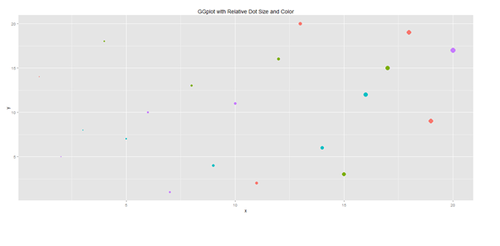```# Scatterplot with regression line. Use the “diamonds” dataset, which is a data frame with
# 53,940 rows and 10 variables ()
# describe(diamonds)
```
```# Use Case-Studies: https://umich.instructure.com/courses/38100/files/folder/Case_Studies
attach(data1)
# plot variables: DIVYEAR momint dadint momclose depression livewithmom gethitched
```
```set.seed(110)
# par(mfrow=c(1,2))
data.2 <- diamonds[sample(nrow(diamonds), 500), ]
plot.2 <- qplot(price, depth, data = data.2, geom = c("point", "smooth"), method = "lm")
plot.3 <- qplot(carat, price, data=data.2, geom=c("point", "smooth"), span=0.4)
print(plot.2); print(plot.3)
```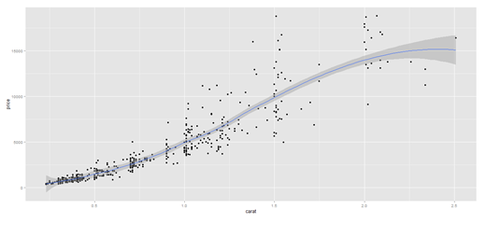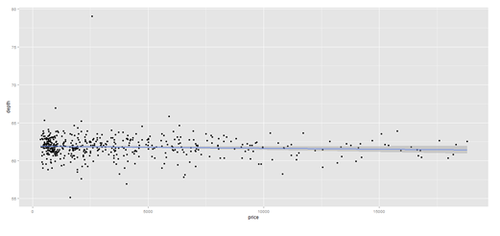### Barplots

```x <- matrix(runif(50), ncol=5, dimnames=list(letters[1:10], LETTERS[1:5]))
barplot(x[1:4,], ylim=c(0, max(x[1:4,])+0.3), beside=TRUE, legend.text = letters[1:4],
args.legend = list(x = "topleft"))
text(labels=round(as.vector(as.matrix(x[1:4,])),2), x=seq(1.5, 21, by=1) + sort(rep(c(0,1,2,3,4), 4)), y=as.vector(as.matrix(x[1:4,]))+0.1)
```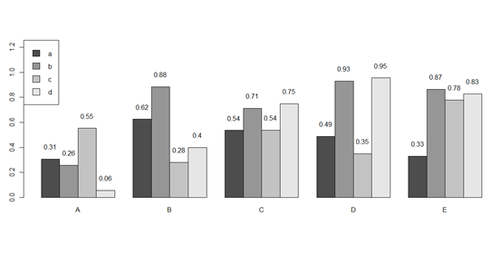```# to put error bars on barplot:
```
```# 10 rows (a, b, c, …):
bar <- barplot(m <- rowMeans(x) * 10, ylim=c(0, 10))
stdev <- sd(t(x))
arrows(bar, m, bar, m + stdev, length=0.15, angle = 90)
```

```# Case_04_ChildTrauma
attach(data1)
# plot data
data2 <- data1[,-5]   # remove the 5th columns text
data1 <- data2[,-5]   # remove the 6th columns text
# or data1 <- data1[,c(-5,-6)]
```
```data2 <- as.data.frame(data1)
Blacks <- data2[which(data2\$\\$\$race=="black"),]
Other <- data2[which(data2\$\\$\$race=="other"),]
Hispanic <- data2[which(data2\$\\$\$race=="hispanic"),]
White <- data2[which(data2\$\\$\$race=="white"),]
```
```A <- c(mean(Blacks\$\\$\$age), mean(Blacks\$\\$\$service))
#colnames(A) <- c("age "," service ")
B <- c(mean(Other\$\\$\$age), mean(Other\$\\$\$service))
C <- c(mean(Hispanic\$\\$\$age), mean(Hispanic\$\\$\$service))
D <- c(mean(White\$\\$\$age), mean(White\$\\$\$service))
```
```x <- cbind(A, B, C, D)
```
```bar <- barplot(x[1:2,], ylim=c(0, max(x[1:2,])+2.0), beside=TRUE,
legend.text = c("age","service") ,  args.legend = list(x = "right"))
text(labels=round(as.vector(as.matrix(x[1:2,])),2), x=seq(1.4, 21, by=1.5), #y=as.vector(as.matrix(x[1:2,]))+0.3)
```

y=11.5)

```m <- x; stdev <- sd(t(x))
arrows(bar, m, bar, m + stdev, length=0.15, angle = 90)
```

```barplot(as.matrix(data1[1:4,]), ylim=c(0, max(data1[1:4,])+0.3), beside=TRUE, legend.text = data1[1:4,1], args.legend = list(x = "topleft"))
text(labels=round(as.vector(as.matrix(data1[1:4,])),2), x=seq(1.5, 21, by=1), y=as.vector(as.matrix(data1[1:4,]))+0.1)
```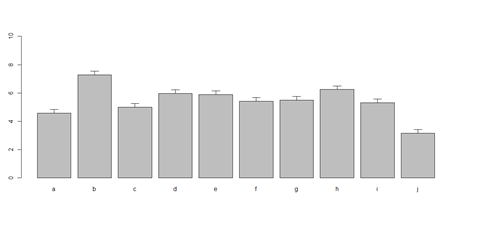```# Columns (A, B, C, D, E):
bar <- barplot(m <- colMeans(x) * 5, ylim=c(0, 5))
stdev <- sd(t(x))
arrows(bar, m, bar, m + stdev, length=0.15, angle = 90)
```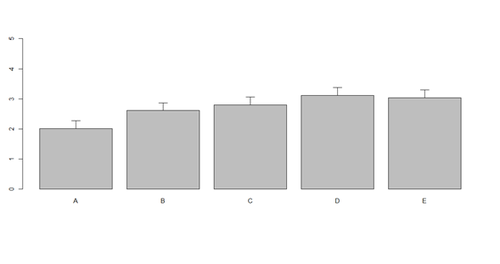### Histograms and Density Plots

```hist(x, freq=TRUE, breaks=10)
```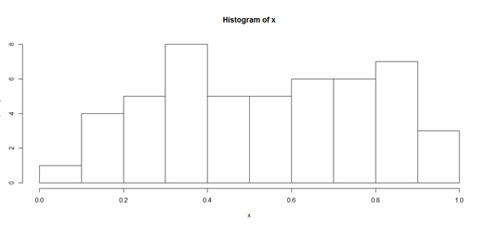```plot(density(x), lwd = 10, col="green")
```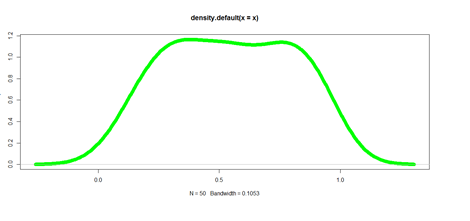### Pie Chart

```# first , “A”, and second, “B”, columns
par (mfrow=c(1,2))
pie(x[,1], col=rainbow(length(x[,1]), start=0.1, end=0.8), clockwise=TRUE)
```
```pie(x[,1], col=rainbow(length(x[,1]), start=0.1, end=0.8), clockwise=TRUE)
```
```pie(x[,2], col=rainbow(length(x[,2]), start=0.1, end=0.8), clockwise=TRUE)
legend("topleft", legend=row.names(x), cex=1.3, bty="n", pch=15, pt.cex=1.8, col=rainbow(length(x[,2]), start=0.1, end=0.8), ncol=1)
```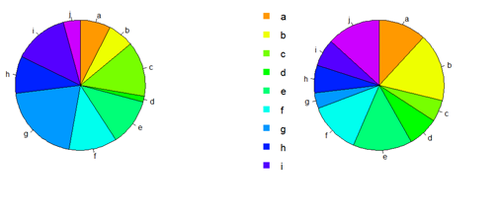```You can export the data:
write.table(x, " ", "data.txt")
# copy-paste it in SOCR Pie chart to generate another Pie view of data
```

### Line Plots Using ggplot

```head(diamonds)
```
 Carat Cut Color Clarity Depth Table Price X Y Z 1 0.23 Ideal E SI2 61.5 55 326 3.95 3.98 2.43 2 0.21 Premium E SI1 59.8 61 326 3.89 3.84 2.31 3 0.23 Good E VS1 56.9 65 237 4.05 4.07 2.31 4 0.29 Premium I VS2 62.4 58 334 4.2 4.23 2.63 5 0.31 Good J SI2 63.3 58 335 4.34 4.35 4.75 6 0.24 VeryGood J VVS2 62.8 57 336 3.94 3.96 2.48
```plot.2 <- ggplot(diamonds, aes(carat, price, group=cut, color=cut)) + geom_line()
print(plot.2)
```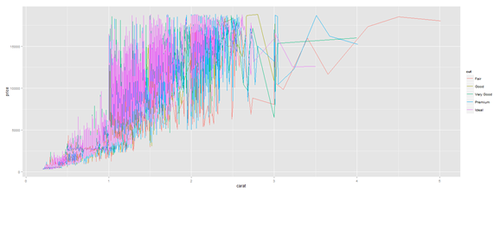```plot.2 <- ggplot(data1, aes(age, service, group=race, color=race)) + geom_line()
print(plot.2)
```

```# Faceting plot (geometrically, faceting (or facetting) is the process of removing parts of a polygon, polyhedron or polytope, without creating any new vertices)
plot.3 <- ggplot(diamonds, aes(carat, price)) + geom_line(aes(color=cut), size=1) +
facet_wrap(~cut, ncol=1)
print(plot.3)
```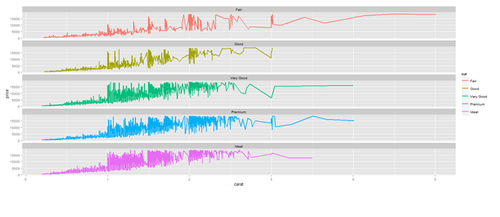### Barplots with ggplot

```plot.4 <- ggplot(diamonds, aes(cut, fill=cut)) + geom_bar() + facet_grid(. ~ clarity)
print(plot.4)
```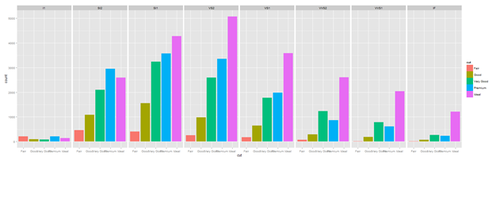```New_var <- service+rnorm(1000, 0,1)
data1\$\\$\$New_var <- int(New_var)
plot.4 <- ggplot(data1, aes(race, fill= traumatype)) + geom_bar() + facet_grid(. ~ New_var)
print(plot.4)

plot.4a <- ggplot(diamonds, aes(color, price/carat, fill=color)) + geom_boxplot()
print(plot.4a)

<center>[[Image:SMHS_SciVisualization20.png|500px]] </center>

==='"`UNIQ--h-11--QINU`"'Jitter plot===

plot.5 <- ggplot(diamonds, aes(color, price/carat)) + geom_jitter(alpha = I(1 / 2), aes(color=color))
print(plot.5)

<center>[[Image:SMHS_SciVisualization21.png|500px]] </center>

==='"`UNIQ--h-12--QINU`"'Density Plots===

plot.6 <- ggplot(diamonds, aes(carat, size=2)) + geom_density(aes(color = cut))
print(plot.6)

<center>[[Image:SMHS_SciVisualization22.png|500px]] </center>

plot.6 <- ggplot(data1, aes(age, size=2)) + geom_density(aes(color = traumatype))
print(plot.6)

plot.7 <- ggplot(diamonds, aes(carat, size=2)) + geom_density(aes(fill = color))
print(plot.7)

<center>[[Image:SMHS_SciVisualization23.png|500px]] </center>

plot.8 <- ggplot(diamonds, aes(x=carat, size=1)) + geom_histogram(aes(y = price), binwidth=0.2) + geom_density()
print(plot.8)

<center>[[Image:SMHS_SciVisualization24.png|500px]] </center>

plot.8a <- ggplot(diamonds, aes(x=carat, size=1)) + geom_histogram(aes(y = price), stat="identity") + geom_density()
print(plot.8a)

<center>[[Image:SMHS_SciVisualization25.png|500px]] </center>

==='"`UNIQ--h-13--QINU`"'Heatmaps===

# Generating Dendogram Association Heatmap Plot (Genotype vs. Imaging phenotype)
# http://stat.ethz.ch/R-manual/R-patched/library/stats/html/heatmap.html
# http://www.ncbi.nlm.nih.gov/pmc/articles/PMC4005931/

require(graphics)
require(grDevices)
library(gplots)

MCI_Data <- MCI_Associations_Data
NC_Data <- NC_Associations_Data

MCI_mat  <- as.matrix(MCI_Data); class(MCI_mat) <- "numeric"
NC_mat  <- as.matrix(NC_Data); class(NC_mat) <- "numeric"

# set up the rol (rc) and column (cc) colors for each cohort
rcMCI <- rainbow(nrow(MCI_mat), start = 0, end=1.0); ccMCI<-rainbow(ncol(MCI_mat),start=0,end=1.0)
rcNC <- rainbow(nrow(NC_mat), start = 0, end = 1.0); ccNC<-rainbow(ncol(NC_mat), start = 0, end = 1.0)

# set up 1x3 graph display - par (mfrow=c(1,3)) – does not work with ‘heatmap’
hvMCI <- heatmap(MCI_mat, col = cm.colors(256), scale = "column", RowSideColors = rcMCI, ColSideColors = ccMCI, margins = c(2,2), main="MCI Cohort SNP-ROI_volume Association (p_values)")
hvNC <- heatmap(NC_mat, col = cm.colors(256), scale = "column", RowSideColors = rcNC, ColSideColors = ccNC, margins = c(2,2), main="NC Cohort SNP-ROI_volume Association (p_values)")

<center>[[Image:SMHS_SciVisualization26.png|500px]] </center>

# Alternatively, we can use the R package gplots
install.packages("gplots")
library(gplots)
## col dendrogram plotted and col reordering done.
## A more decorative heatmap, with z-score scaling along columns

<center>[[Image:SMHS_SciVisualization27.png|500px]] </center>

==='"`UNIQ--h-14--QINU`"'Correlation Plots===

The <b>corrplot</b> package is a graphical display of a correlation matrix and confidence intervals, with some tools for matrix reordering. There are seven visualization methods (parameter method) in corrplot package, named   "circle", "square", "ellipse", "number", "shade", "color", "pie".
# install.packages("corrplot")
library(corrplot)
M <- cor(NC_Associations_Data)

<center>[[Image:SMHS_SciVisualization28.png|500px]] </center>

==='"`UNIQ--h-15--QINU`"'Hyperbolic Visualization===

•	URL: http://socr.umich.edu/html/Navigators.html

•	Tools:

<blockquote>o	Java/Jar applet: http://socr.umich.edu/html/navigators/HW/jars/SOCR_HW_Viewer.jar</blockquote>

<blockquote>o	JavaScript: http://socr.umich.edu/html/navigators/D3/SOCR_D3_Viewer.html (JSON)</blockquote>

•	Data Format

<blockquote>o	XML data: http://socr.umich.edu/html/navigators/HW/SOCR_HyperTree.xml</blockquote>

<blockquote>o	JSON data: http://socr.umich.edu/html/navigators/D3/xml/SOCR_HyperTree.json</blockquote>

•	D3 Visualization

<blockquote>o	E:\Ivo.dir\Research\UMichigan\Education_Teaching_Curricula\2015_2016\HS_853_Fall_2015\Modules_docx\Tools\TreeViewer_JS</blockquote>

<blockquote>o	treeJS.json</blockquote>

<blockquote>o	flareD3.json</blockquote>

<center>[[Image:SMHS_SciVisualization29.png|400px]] </center>

<center>[[Image:SMHS_SciVisualization30.png|400px]] </center>

•	URL: https://github.com/mbostock/d3/wiki/Gallery

•	Source code: https://github.com/mbostock/d3

<center>[[Image:SMHS_SciVisualization31.png|400px]] </center>

==='"`UNIQ--h-16--QINU`"'Motion Charts===

•	Video: http://www.socr.ucla.edu/SOCR_MotionCharts/SOCR_HTML5_MotionChart_Video2.gif

•	Java: http://www.socr.ucla.edu/SOCR_MotionCharts/

•	HTML5: http://socr.umich.edu/HTML5/MotionChart/

•	Activities: http://wiki.socr.umich.edu/index.php/SOCR_MotionCharts

<center>[[Image:SMHS_SciVisualization32.png|500px]] </center>

==='"`UNIQ--h-17--QINU`"'1D/2D/3D signal/area/volume/surface/model/atlas visualization===

• 1D: (See R/SOCR Visualization tools above)

• 2D: http://imagej.nih.gov/ij/

• 3D: http://socr.umich.edu/HTML5/BrainViewer/

<b>Supported File Formats:</b>
Volumes (.nii / .nii.gz / .img&.hdr / .mgh / .mgz / .nrrd)
Shapes (.dx / .vtk / .stl / FreeSurfer)
Fibers (.trk)

<center>[[Image:SMHS_SciVisualization33.png|500px]] </center>

==='"`UNIQ--h-18--QINU`"'Trees and Graphs===

• Trees/Hierarchies and general Graphs

# Install and load the APE package, needed for the phylogenetic tree rendering (as.phylo)
# install.packages("ape")
library("ape")

# Data: 02_Nof1_Data.csv

<center>
{| class="wikitable" style="text-align:center; width:35%" border="1"
|-
mydata1
|-
|||ID||Day||Tx||SelfEff||SelfEff25||WPSS||SocSuppt||PMss||PMss3||PhyAct
|-
|1||1||1||1||33||8||0.97||5.00||4.03||1.03||53
|-
|2||1||2||1||33||8||-0.17||3.87||4.03||1.03||73
|-
|3||1||3||0||33||8||0.81||4.84||4.03||1.03||23
|-
|4||1||4||0||33||8||-0.41||3.62||4.03||1.03||36
|-
|5||1||5||1||33||8||0.59||4.62||4.03||1.03||21
|-
|6||1||6||1||33||8||-1.16||2.87||4.03||1.03||0

|}
</center>

Clustering

hc = hclust(dist(data.1), 'ave')
# the agglomeration method can be specified "ward.D", "ward.D2", "single","complete", "average" (= UPGMA), "mcquitty" (= WPGMA),"median" (= WPGMC) or "centroid" (= UPGMC)

# (3) Plot clustering diagram
par (mfrow=c(1,1))
# very simple dendrogram
plot(hc)

<center>[[Image:SMHS_SciVisualization34.png|500px]] </center>

require(graphics)
(x <- identify(hc)) ##  Terminate with 2nd mouse button !!
identify(hc, <mark>function(k)</mark> print(table(data.1[k,5])))

You can now cut the tree into branches. You can split the tree into 2 groups, by setting the number of cuts with the k=2 parameter, or by specifying height to cut the tree at (?cutree):

k- an integer scalar or vector with the desired number of groups

h- numeric scalar or vector with heights where the tree should be cut

cutree(hc, k = 2)
# alternatively specify the height, which is, the value of the criterion associated with the clustering method
# for the particular agglomeration.
cutree(hc, h= 50) # cut at h=50
table(cutree(hc, h= 50)) # cluster distribution
# To identify the number of cases for varying number of clusters we can combine calls to cutree and table
# in a call to <b>sapply</b> -- to see the sizes of the clusters for 2≤ k≤10 cluster-solutions:
# numbClusters=5;
myClusters = sapply(2:10,function(numbClusters)table(cutree(hc, numbClusters)))
names(myClusters) <- paste("Number of Clusters=", 2:10, sep = "")
myClusters
#To see which SubjectIDs are in which clusters:
groups.10 <- cutree(hc, k = 10)
sapply(unique(groups.10),function(g)data.1\$\\$\$ID[groups.10 == g])
#To see which Treatments (Tx) are in which clusters:
groups.2 <- cutree(hc, k = 2)
sapply(unique(groups.2),function(g)data.1\$\\$\$Tx[groups.2 == g])
# drill down deeper
table(groups.2, data.1\$\\$\$Tx)
# For a small number of observations, we can often interpret the cluster solution directly by looking
# at the labels of the observations that are in each cluster.
# This is hard for larger data sets. To characterize clusters we can look at cluster summary statistics,
# like the median, of the variables that were used to perform the cluster analysis broken down
# by the groups that the cluster analysis identified.
```

The aggregate function will compute stats (e.g., median) on many variables simultaneously.

To look at the median values for the variables we've used in the cluster analysis, broken up by the cluster groups:

```aggregate(data.1, list(groups.10),median) # may have to shrink data.1 prior to clustering!
# data.2 <- data.1[,-c(1,3)]  # Remove ID and Tx variables?
aggregate(data.2, list(groups.2),median) # for only 2 clusters
```
 Group ID Day Tx SelfEff SelfEff25 WPSS SocSuppt PMss PMss3 PhyAct 1 1 14 16 0 20 -5 -0.040 2.995 3.275 0.275 41 2 2 16 15 1 25 0 0.025 3.280 3.360 0.360 104
```table(groups.2, data.1\$\\$\$<u><b>PhyAct</b></u>)

# library("Hmisc")

describe(data.1\$\\$\$PhyAct)
```
```# It’s useful to add the numbers of observations in each group (aggregate returns a data frame,
# that can be manipulated)
```
```df.2 <- aggregate(data.1, list(groups.2),median)
```
```data.frame(Cluster= df.2[,1], Freq=as.vector(table(groups.2)), df.2[,-1])
```
 Cluster Freq ID Day Tx SelfEff SelfEff25 WPSS SocSuppt PMss PMss3 PhyAct 1 1 570 14 16 0 20 -5 -0.040 2.995 3.275 0.275 41 2 2 330 16 15 1 25 0 0.025 3.280 3.360 0.360 104

### Publications

• This paper examines nasal and bronchial tissue cultures as appropriate in vitro models for the assessment of smoking-induced adverse effects in the respiratory system (doi: 10.1177/1091581814551647), using “hclust” package. No data.

• This paper classified subtypes of gastric cancer based on epidemiologic and histologic and gene expression data. These new classifications of gastric cancer have implications for improving our understanding of disease biology and identification of unique molecular drivers for each gastric cancer subtype (doi: 10.1158/1078-0432.CCR-10-2203).

Repeat the clustering

```# using centroids and squared Euclidean distance
# cut the tree into 10 clusters and reconstruct the upper part of the tree from the cluster centers.
hc <- hclust(dist(data.1), "cen")
mem <- cutree(hc, k = 10)
cent <- NULL
for(k in 1:10){
cent <- rbind(cent, colMeans(data.1[mem == k, , drop = FALSE]))
}
```
```hc1 <- hclust(dist(cent), method = "cen", members = table(mem))
```
```opar <- par(mfrow = c(1, 2))
plot(hc,  labels = FALSE, hang = -1, main = "Original Tree")
plot(hc1, hang = -1, main = "Re-start from 10 clusters")
par(opar)
```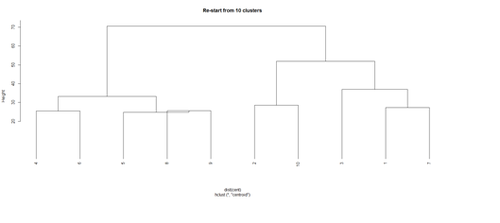Identify subjects within each of the 10 classes

```rect.hclust(hc, h=10)
```
```# To save the cluster numbers to a new variable in the dataset, use the cutree function.
# data.1\$\\$\$clusterID <- cutree(hc, 10)
data.1\$\\$\$clusterID <- cutree(hc, 10)
```
 ID Day Tx SelfEff SelfEff25 WPSS SocSuppt PMss PMss3 PhyAct CluserID 1 1 1 1 33 8 0.97 5.00 4.03 1.03 53 1 2 1 2 1 33 8 -0.17 3.87 4.03 1.03 73 1 3 1 3 0 33 8 0.81 4.84 4.03 1.03 23 2 4 1 4 0 33 8 -0.41 3.62 4.03 1.03 36 2 5 1 5 1 33 8 0.59 4.62 4.03 1.03 21 2 6 1 6 1 33 8 -1.16 2.87 4.03 1.03 0 2

Phylogenetic tree diagram

```# library("ape")
plot(as.phylo(hc1), use.edge.length = TRUE, type = "fan")
plot(as.phylo(hc), use.edge.length = TRUE, type = "fan", tip.color = hsv(runif(15, 0.65, 0.95), 1, 1, 0.7), label.offset = 1, cex = log(data.1\$\\$\$ID, 10), col = "red")

<center>[[Image:SMHS_SciVisualization36.png|500px]] </center>

<center>[[Image:SMHS_SciVisualization37.png|500px]] </center>

==='"`UNIQ--h-20--QINU`"'Hands-on Activity (Health Behavior Risks)===

# Classify the cases using these variables:  "AGE_G"    "SEX"      "RACEGR3"  "IMPEDUC"  "IMPMRTL"
#	"EMPLOY1"  "INCOMG"  "CVDINFR4" "CVDCRHD4" "CVDSTRK3" "DIABETE3" "RFSMOK3"
#	"FRTLT1"   "VEGLT1"
data.raw <- data.2[,-c(1,14,17)]

# Does the classification match either of these:
#	TOTINDA (Leisure time physical activities per month, 1=Yes, 2=No, 9=Don’t know/Refused/Missing)
#	RFDRHV4 (Heavy alcohol consumption, 1=No, 2=Yes, 9=Don’t know/Refused/Missing)

hc = hclust(dist(data.raw), 'ave')
# the agglomeration method can be specified "ward.D", "ward.D2", "single", "complete", "average" (= UPGMA), "mcquitty" (= WPGMA), "median" (= WPGMC) or "centroid" (= UPGMC)

# (3) Plot clustering diagram
par (mfrow=c(1,1))
# very simple dendrogram
plot(hc)

summary(data.2\$\\$\$TOTINDA); summary(data.2\$\\$\$RFDRHV4)

cutree(hc, k = 2)
# alternatively specify the height, which is, the value of the criterion associated with the
# clustering method for the particular agglomeration -- cutree(hc, h= 10)

table(cutree(hc, h= 10)) # cluster distribution

# To identify the number of cases for varying number of clusters we can combine calls to cutree and table
# in a call to sapply -- to see the sizes of the clusters for 2≤ k≤10 cluster-solutions:
# numbClusters=4;
myClusters = sapply(2:5,function(numbClusters)table(cutree(hc, numbClusters)))
names(myClusters) <- paste("Number of Clusters=", 2:5, sep = "")
myClusters

#To see which SubjectIDs are in which clusters:
table(cutree(hc, k=2))
groups.k.2 <- cutree(hc, k = 2)
sapply(unique(groups.k.2),function(g)data.2\$\\$\$ID[groups.k.2 == g])
```
```#To see which TOTINDA (Leisure time physical activities per month, 1=Yes, 2=No, 9=Don’t
# 	know/Refused/Missing) & whch RFDRHV4 are in which clusters:
groups.k.3 <- cutree(hc, k = 3)
sapply(unique(groups.k.3),function(g)data.2\$\\$\$TOTINDA [groups.k.3 == g])
sapply(unique(groups.k.3),function(g)data.2\$\\$\$RFDRHV4[groups.k.3 == g])
```
```# Perhaps there are intrinsically 3 groups here e.g., 1, 2 and 9 …
groups.k.3 <- cutree(hc, k = 3)
sapply(unique(groups.k.3),function(g)data.2\$\\$\$TOTINDA [groups.k.3 == g])
sapply(unique(groups.k.3),function(g)data.2\$\\$\$RFDRHV4 [groups.k.3 == g])
```
```# Note that there is quite a dependence between the outcome variables …
plot(data.2\$\\$\$RFDRHV4, data.2\$\\$\$TOTINDA)
```
```# drill down deeper
table(groups.k.3, data.2\$\\$\$RFDRHV4)
```
```# To characterize clusters we can look at cluster summary statistics,
# like the median, of the variables that were used to perform the cluster analysis broken down
# by the groups that the cluster analysis identified. The aggregate function will compute stats
# (e.g., median) on many variables simultaneously. To look at the median values for the variables
# we've used in the cluster analysis, broken up by the cluster groups:
aggregate(data.2, list(groups.k.3),median)
```

## See Next

• Next See the Complex Network Visualization section
• Back to the Scientific Methods for Health Sciences EBook ToC/